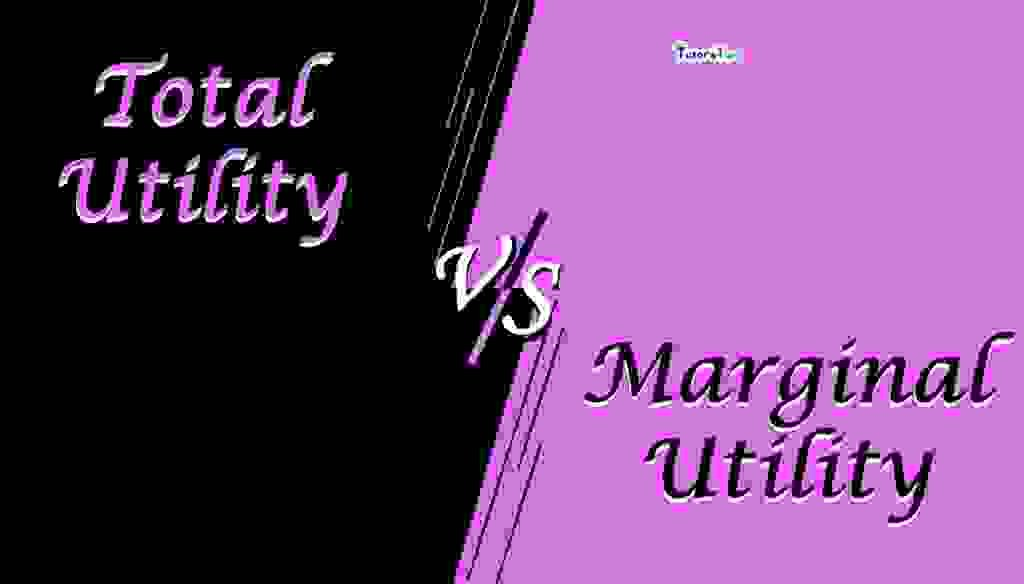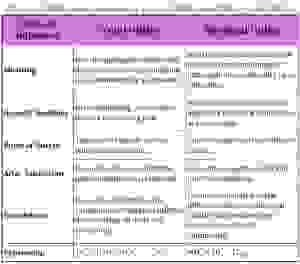# Difference between Total Utility and Marginal UtilityThe main difference between Total Utility and Marginal Utility is that the total utility refers to the satisfaction derived by a consumer from the consumption of a certain amount of a commodity whereas Marginal Utility refers to the additional satisfaction derived by the consumer from consumption of one more unit of a commodity.

To understand its difference, first, we have to know the meaning of both terms:

## Meaning of Total Utility:

Total Utility refers to the total satisfaction obtained from the consumption of all possible units of a commodity. For example, if consumption of one apple gives a person satisfaction of 10 units and consumption of another apple gives the satisfaction of 8 units, the total satisfaction from two apples will be (10+8) = 18 units. If one more apple gives him the satisfaction of 6 units, then total satisfaction becomes (10+8+6)=24 units.

TUn = U1+U2+U3+………..+Un

Where,

TU = Total utility from n units of a given commodity

n = number of units consumed

U1, U2, U3 ………….U= utility from 1st, 2nd, 3rd to an nth unit of commodity.

## Meaning of Marginal Utility:

Marginal Utility refers to the additional utility derived from the consumption of one more unit of a given commodity in a given time period.

As in the above example, the total utility increases from 18 to 24 units after the consumption of 3rd apple. Thus, an additional 6 units are the marginal utility-driven from the 3rd unit of apple.

MU can be calculated as

MUn = TUn – TUn-1

Where MU= marginal utility from an nth unit

TUn = Total utility from n units

TUn-1 = Total utility from n-1 units

In simple words, MU is the change in total utility when more unit of a given commodity is consumed.

Therefore,

“MU= Change in TU/ Change in units consumed”

## Chart of Difference between Total Utility and Marginal Utility

Basis of Difference

Total Utility

Marginal Utility

#### Meaning

It is the aggregate satisfaction derived from the consumption of a commodity by a consumer It is the additional satisfaction derived from consuming an extra unit of a commodity by a consumer.

#### General tendency

In the beginning, it increases but at a decreasing rate. It tends to diminish as more and more units of a commodity are consumed

#### Point of Satiety

The point of satiety arrives when it is maximum. Point of satiety arrives where it is zero.

#### After Saturation

It tends to decrease after the point of saturation is reached. It becomes negative after the point of saturation.

#### Calculation

It can be calculated as the summation of marginal utilities derived from all units of a commodity It can be calculated as the difference between the total utilities derived from two successive units of a commodity.

#### Expression

TUn = U1+U2+U3+………..+Un MUn = TUn – TUn-1

## Download the chart: –

If you want to download the chart please download the following image and PDF file:-Difference between Total Utility and Marginal UtilityDifference between Total Utility and Marginal Utility

## Conclusion: –

Thus,  Total Utility and Marginal Utility are the types of term Utility. These both are used to analyze the consumers’ preferences while purchasing in a market.

Thanks Please share with your friends

Comment if you have any question.

Check out Business Economics Books @ Amazon.in